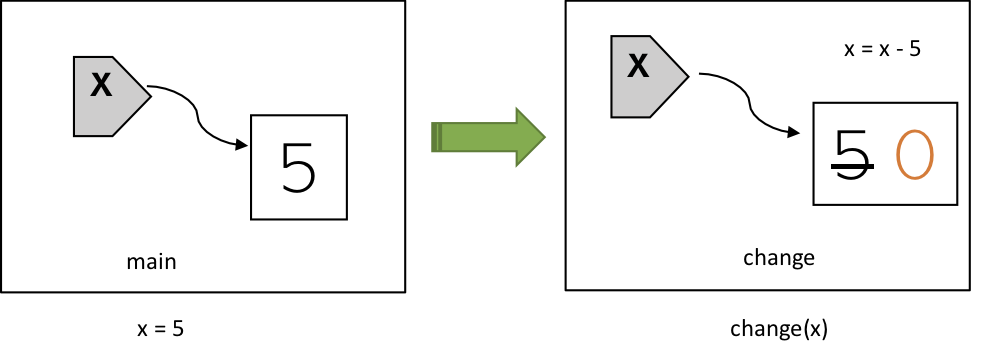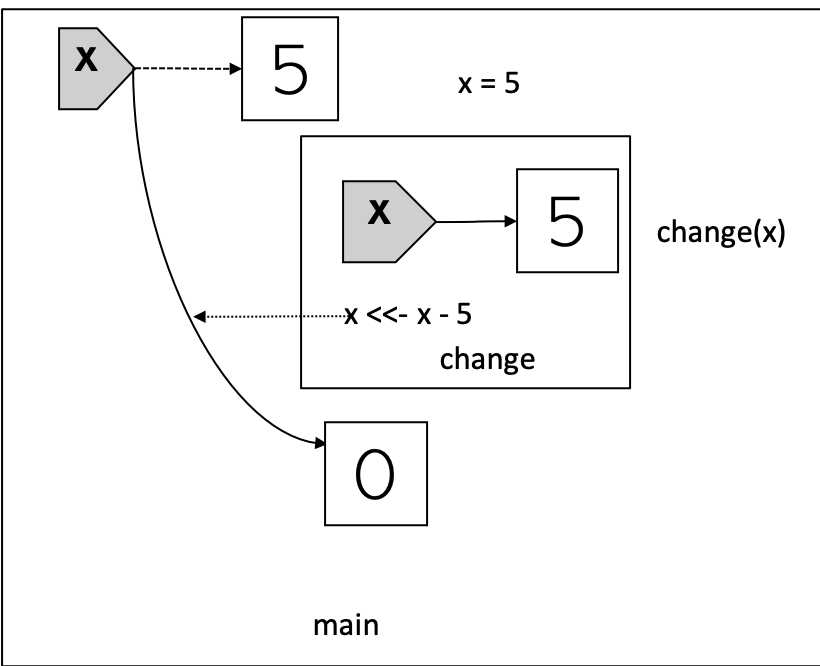by Guangming Lang

### Categories

• r

If you have been following this R Functional Programming (RFP) series1, you know by now we have discussed:

In summary, we have the following facts about the R language:

• Variable assignment binds symols to objects.
• Reassignment changes the binding. It doesn’t modify data.
• Mutability is a property of objects. It has nothing to do with variables or variable assignment.
• Copy-n-modify is everywhere.

To better understand these facts, let’s walk through two examples. The first example consists of the following code block:

What will happen if we call main()?

1. x will be bound to 5.
2. 5 and its memory address will be printed.
3. change(x) will not change the value of x.
4. 5 and the same address will be printed.

Let’s call it now:

The output confirms our reasoning. When the line change(x) is executed, a new environment is created, then the value 5 is copied into this new environment and bound to a new variable x2. When the line x = x - 5 is run, R looks up the value 5 of this new x from this new environment, carries out the subtraction and rebinds x to the result 0. When the program steps out of the function change(), everything (x, 0, 5) inside of this new environment will be garbage collected. I want to point out that the original x in main() is never modified. See the following diagram:## <<- Reassigns Variables in Outer Scope

Now let’s look at the second example:

This example differs from the first one at two places:

• change() is defined inside of main().
• <<- is used instead of <- or = (x <<- x - 5 instead of x = x - 5).

The <<- operator is usually only used in functions. While operators <- and = perform variable assignment inside the environment (or scope) where they are evaluated, <<- causes a search through parent environments for the variable being assigned. If such a variable is found (and its binding is not locked) then its value is redefined, otherwise assignment takes place in the global environment. I’d like to point out that like <- and =, <<- also only changes the binding and doesn’t modify data.

In this example:

• change() defines an inner scope and main() defines an outer scope.
• because of <<-, change(x) changes the value of x defined in the outer scope.

When we call main(), the following will happen:

1. change will be bound to function(x) { x <<- x - 5 ...} in the outer scope.
2. x will be bound to 5 in the outer scope.
3. 5 and its memory address will be printed.
4. change(x) will change the value of outer x to 0 and print 5 and a different location.
5. 5 and yet another different location will be printed.

Let’s call main() and look at the output:

The 2nd line of output is particularly interesting. You’re probably expecting it to print 0, but that wouldn’t be correct. When the line change(x) is executed, a new environment is created, then the value 5 is copied into this new environment and bound to a new variable x2. When the line x <<- x - 5 is run, R looks up the value 5 of this new x from this new environment, carries out the subtraction and rebinds the x in the outer scope to the result 0. Notice that the inner variable x is still bound to 5. When R executes the line print(paste("do work and get:", x, "at addr", pryr::address(x))), it looks up the value and address of the inner x, and hence prints 5. Of course, because this 5 is a copy of the outer 5, it is a different 5 and hence has a different memory address. See the following diagram:To summarize, here’re the facts about <<-:

• it’s usually only used in functions.
• it reassigns outer variables by changing its binding.
• it never modifies data directly.

Here’s an example of how <<- can be used in real world:

Mortgage Payment Schedule Calculator

1. If you haven’t, I recommend you to read the linked posts first.

2. different from the x in main() 2